International
Tables for
Crystallography
Volume B
Reciprocal space
Edited by U. Shmueli

International Tables for Crystallography (2010). Vol. B, ch. 1.3, p. 41   | 1 | 2 |

## Section 1.3.2.5.8. Multiplication and convolution

G. Bricognea

aGlobal Phasing Ltd, Sheraton House, Suites 14–16, Castle Park, Cambridge CB3 0AX, England, and LURE, Bâtiment 209D, Université Paris-Sud, 91405 Orsay, France

#### 1.3.2.5.8. Multiplication and convolution

| top | pdf |

Multiplier functionsfor tempered distributions must be infinitely differentiable, as for ordinary distributions; furthermore, they must grow sufficiently slowly asto ensure thatfor alland that the map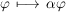is continuous for the topology of. This leads to choosing for multipliers the subspaceconsisting of functionsof polynomial growth. It can be shown that if f is in, then the associated distributionis in(i.e. is a tempered distribution); and that conversely if T is inis infor all.

Corresponding restrictions must be imposed to define the space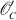of those distributions T whose convolutionwith a tempered distribution S is still a tempered distribution: T must be such that, for allis in; and such that the map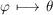be continuous for the topology of. This implies that S is `rapidly decreasing'. It can be shown that if f is in, then the associated distributionis in; and that conversely if T is in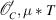is infor all.

The two spacesandare mapped into each other by the Fourier transformation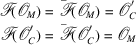and the convolution theorem takes the form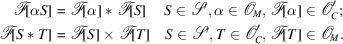The same identities hold for. Taken together with the reciprocity theorem, these show thatandestablish mutually inverse isomorphisms betweenand, and exchange multiplication for convolution in.

It may be noticed that most of the basic properties ofandmay be deduced from this theorem and from the properties of δ. Differentiation operatorsand translation operatorsare convolutions withand; they are turned, respectively, into multiplication by monomials(the transforms of) or by phase factors(the transforms of).

Another consequence of the convolution theorem is the duality established by the Fourier transformation between sections and projections of a function and its transform. For instance, in, the projection ofon the x, y plane along the z axis may be written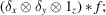its Fourier transform is then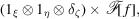which is the section of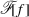by the plane, orthogonal to the z axis used for projection. There are numerous applications of this property in crystallography (Section 1.3.4.2.1.8) and in fibre diffraction (Section 1.3.4.5.1.3).